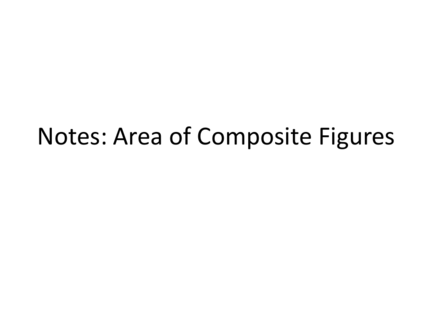# Area of Composite Figures: ExplainedContributed by:This pdf includes the following topics:-
Definition and Strategy
Examples
Applications
1. Notes: Area of Composite Figures
2. I. Definition and Strategy
A composite figure is made up of simple
shapes, such as triangles, rectangles,
trapezoids, and circles. To find the area of a
composite figure, find the areas of the simple
shapes and then add them together.
3. II. Examples
Ex 1a: Find the shaded area. Round to the
nearest tenth, if necessary.
Divide the figure into parts.
area of half circle:
area of triangle:
area of the rectangle: A = bh = 20(14) = 280 mm2
shaded area: 50 + 280 + 84 ≈ 521.1 mm2
4. Ex 1b: Find the shaded area. Round to the
nearest tenth, if necessary.
Divide the figure into parts.
area of parallelogram:
A = bh = 8(5)= 40ft2
area of triangle:
shaded area: 40 + 25 = 65 ft2
5. Ex 1c: Find the shaded area. Round to the
nearest tenth, if necessary.
Area of rectangle:
A = bh = 37.5(22.5)
= 843.75 m2
Area of triangle:
= 937.5 m2
6. III. Examples with Subtraction
Sometimes you might need to subtract a figure.
Ex 2a: Find the shaded area. Round to the nearest
tenth, if necessary.
area of a triangle:
area of the half circle:
Subtract the area of the area of figure:
half circle from the area
234 – 10.125 ≈ 202.2 ft2
of the triangle.
7. Ex 2b: Find the shaded area. Round to the
nearest tenth, if necessary.
area of circle:
A = r2 = (10)2 = 100 cm2
area of trapezoid:
area of figure: 100 –128  186.2 cm2
8. Ex 2c: Find the shaded area. Round to the
nearest tenth, if necessary.
area of circle:
A = r2 = (3)2  28.3 in2
area of square:
A = bh  (4.24)(4.24)  18 in2
area of figure: 28.3 – 18 = 10.3 in2
9. IV. Applications
Ex 3a: A company receives an order for 65 pieces of fabric in
the given shape. Each piece is to be dyed red. To dye 6 in2 of
fabric, 2 oz of dye is needed. How much dye is needed for the
entire order?
To find the area of the shape in square
inches, divide the shape into parts.
The two half circles have the same area as
one circle.
The area of the circle is (1.5)2 = 2.25 in2.
The area of the square is (3)2 = 9 in2.
The total area of the shape is 2.25 + 9 ≈ 16.1 in2.
The total area of the 65 pieces is 65(16.1) ≈ 1044.5 in2.
The company will need 1044.5 ≈ 348 oz of dye
for the entire order.
10. Ex 3b: The lawn that Katie is replacing requires 79 gallons
of water per square foot per year. She wants to replace the
lawn with a xeriscape garden, which only uses 17 gallons of
water per square foot per year. How much water will Katie
save, per year, by planting a xeriscape garden?
Find the area of the figure.
Area of large rectangle = bh =
7.5(28.5) = 213.75 ft2
(𝑏1 +𝑏2 )ℎ 12+18 6
Area of trapezoid= = =90 ft2
2 2
Area of small rectangle = bh = 6(12)= 72 ft2
Area of garden = 213.75+90+72=375.75 ft2
Area times gallons of water 375.75(79) = 29,684.25
Subtract water used
29,684.25 – 6,387.75 = 23,296.5 gallons saved.